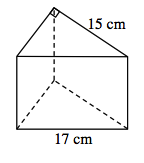### Home > CCG > Chapter Ch10 > Lesson 10.3.5 > Problem10-178

10-178.

A triangular prism has a volume of $600$ cubic cm. The base is a right triangle with a hypotenuse of length $17$ cm and one leg with length $15$ cm.

1. Draw the figure.2. Find the height of the prism.

Use the area of the base and the given volume to calculate the height.

3. Find the surface area of the prism.

4. Find all three angles of the triangle in the base of the prism.

$90º$$\sin a=\frac{8}{17}, \ a=28º$$\sin b=\frac{15}{17}, b=62º$